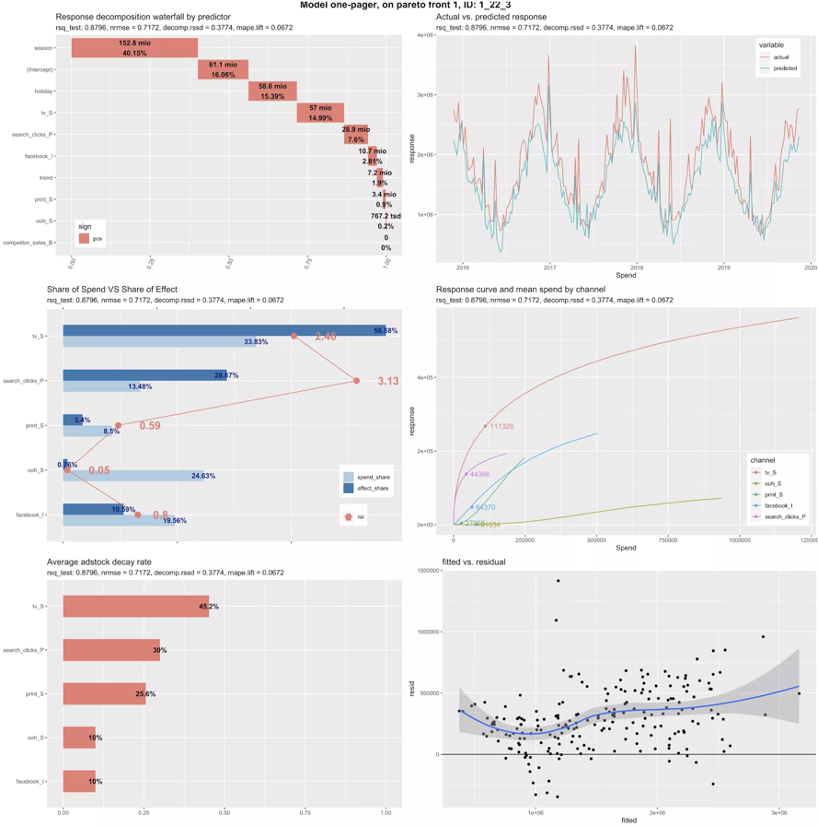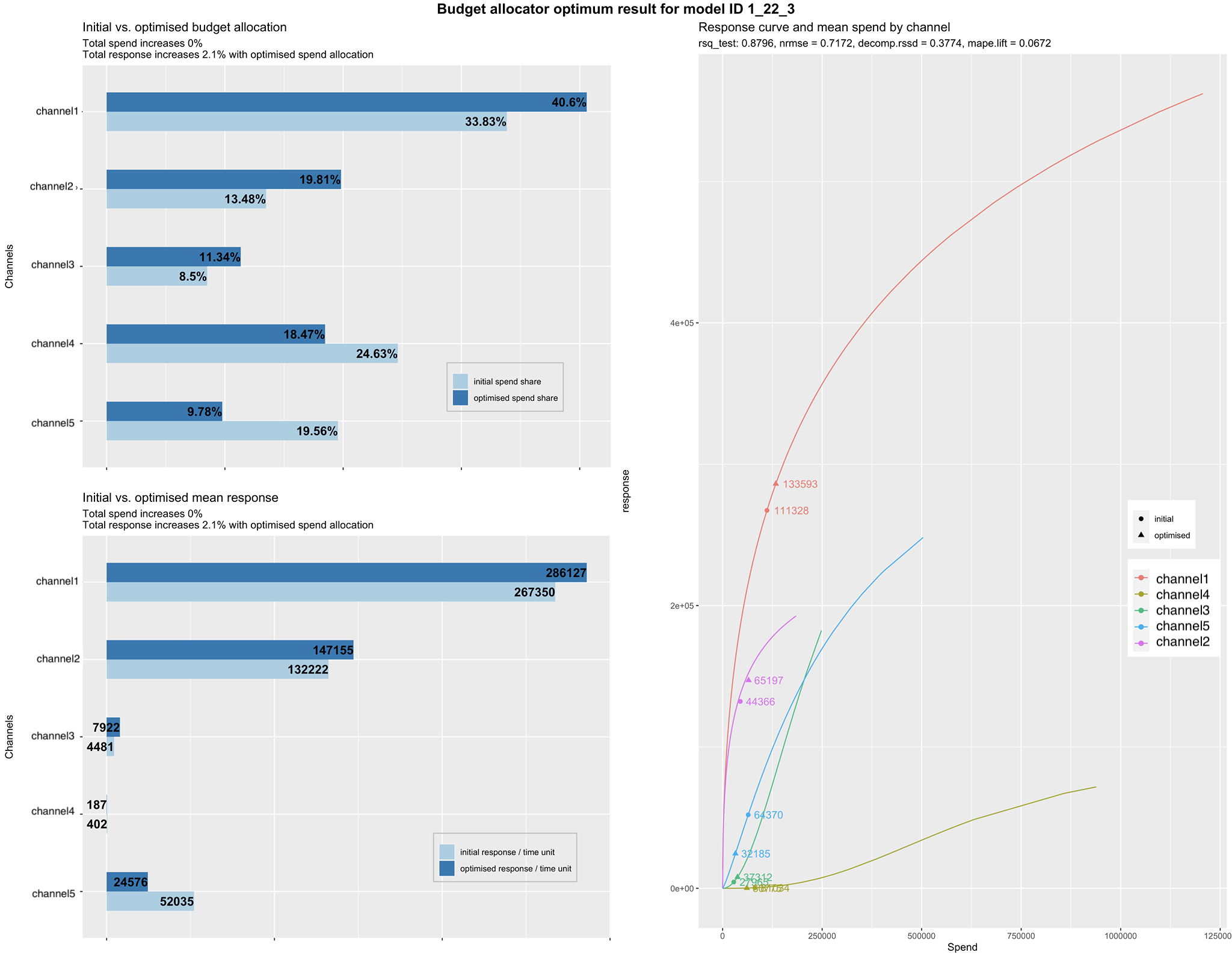# Outputs and diagnostics

The MMM code will automatically generate a set of plots under the folder you specify on the ‘model_output_collect’ object. Each of these plots represents one of the optimal model solutions as a result of the multi-objective optimization Pareto optimal process mentioned in the ‘Automated hyperparameter selection and optimization’ section. Please find below an example of the model output:As you may observe we have 6 different charts above:

1. Response decomposition waterfall by predictor: This chart reflects the percentage of each of the variables effect (Baseline and Media variables + intercept) on the response variable. E.g. If season effect says it's 40.5% that means that 40.5% of the total sales can be attributed to seasonality.
2. Share of spend vs. share of effect: This plot reflects the comparison of the total effect each channel had by means of the decomposition of the coefficients into the response variable divided by the total effect. As well as, the total spend (cost or investment) each channel had and its relative share over total marketing spend. We also plot the return on investment (ROI) each channel had which can give you an idea over the most profitable channels.
3. Average adstock decay rate: This chart represents, on average, what is the percentage decay rate each channel had. The higher the decay rate, the longer the effect in time for that specific channel media exposure.
4. Actual vs. predicted response: This plot shows the actual data for the response variable E.g sales, and how the modeled predicted data for that response variable is capturing the real curve. We aim for models that can capture most of the variance from the actual data and therefore the R-squared is closer to 1 while NRMSE is low.
5. Response curves and mean spend by channel: These are the diminishing returns response curves from hill function. They represent how saturated a channel is and therefore, may suggest potential budget reallocation strategies. The faster the curves reach to an inflection point and to a horizontal/flat slope, the quicker they will saturate with each extra (\$) spent.
6. Fitted vs. residual: This chart shows the relationship between fitted and residual values. A residual value is a measure of how much a regression line vertically misses a data point. A residual plot is typically used to find problems with a regression. Some data sets are not good candidates for regression, such as points at widely varying distances from the line. If the points in a residual plot are randomly dispersed around the horizontal axis, a linear regression model is appropriate for the data; otherwise, a nonlinear model is more appropriate.

Once you have analyzed the optimal model results plots and have chosen your model, you may introduce the model unique ID from the results in the previous section. E.g setting modID = "1_22_3" could be an example of a selected model from the list of best models in 'model_output_collect\$allSolutions' results object. Once you run the budget allocator, results will be plotted and saved under the same folder where the model plots had been saved. The result would look like the following:You may encounter three charts as in the example above:

1. Initial vs. optimized budget allocation: This channel shows the original spend share vs. the new optimized recommended one. If optimized share is greater than original, this would mean you will need to proportionally increase budgets for that channel according to the difference between both shares. And you would reduce budgets in the case spend share would be greater than optimized share.
2. Initial vs. optimized mean response: Similar to the chart above, we have initial and optimized shares, but this time over the total expected response E.g. Sales. The optimized response is the total increase in sales that we are expecting you to have if you switch budgets following the chart we explained above, increasing those with better share for optimized spend and decreasing spend for those with lower optimized spend than the initial.
3. Response curve and mean spend by channel: These again are the diminishing returns response curves from hill function. They represent how saturated a channel is and therefore, may suggest potential budget reallocation strategies. The faster the curves reach to an inflection point and to a horizontal/flat slope, the quicker they will saturate with each extra (\$) spent. The initial mean spend is represented by a circle and the optimized one by the triangle.